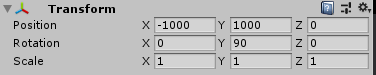# Transform.Rotate not working around certain axes

I am trying to write a missile guidance script using proportional navigation. However, I encountered a problem with the Transform.Rotate function in Unity. When rotating around every axis except the Z-axis (0,0,1), everything works as expected (see video, Unity Transform.Rotate confusion - YouTube if this link doesn’t work try the one below). Only when rotating around the Z-axis nothing happens. So far I have narrowed it down to the -90 degrees X orientation I start the rocket with.I have no idea what is going wrong here. Can someone please help?

``````void FixedUpdate()
{
Vector3 LOS = target.transform.position - transform.position; //Line of sight (Black)
Vector3 LOSGain = LOS.normalized - prevLOS.normalized; //Change in LOS (Red)
float LOSAng = Vector3.Angle(transform.forward, LOS);
Debug.DrawRay(transform.position, LOS, Color.black);
Debug.DrawRay(transform.position, LOSGain*10, Color.red);

Vector3 perpVec = Vector3.Cross(LOS.normalized, LOSGain.normalized); //perpVec is perpindicular to both the LOS and the LOS change

float rotationSpeed = Mathf.Clamp(Mathf.Abs(LOSGain.magnitude * N), 0, maxAngCorr); //rotationspeed = Omega * N (Clamped to maximum rotation speed)

transform.Rotate(perpVec, rotationSpeed); //<--Rotate happens here
Debug.Log("SHOULD BE ROTATING");
Debug.Log("perpVec: " + perpVec);
Debug.Log("rotationSpeed: " + rotationSpeed);
Debug.DrawRay(transform.position, perpVec.normalized * 10, Color.blue);

prevLOS = LOS;
}
``````

I have also tried this parallel code and it has the same problem:

``````Quaternion rot = Quaternion.AngleAxis(rotationSpeed, perpVec.normalized); // rotate with rotationSpeed around perpVec axis.
transform.rotation *= rot;
``````

I have found a solution that avoids the problem by doing the rotation in another way:

``````Vector3 newLookDirection = Quaternion.AngleAxis(rotationSpeed, perpVec) * transform.forward;
transform.LookAt(transform.position + newLookDirection);
``````

However, it would be nice if someone can shine some light on why the other solutions don’t work, but now this one is working flawlessly